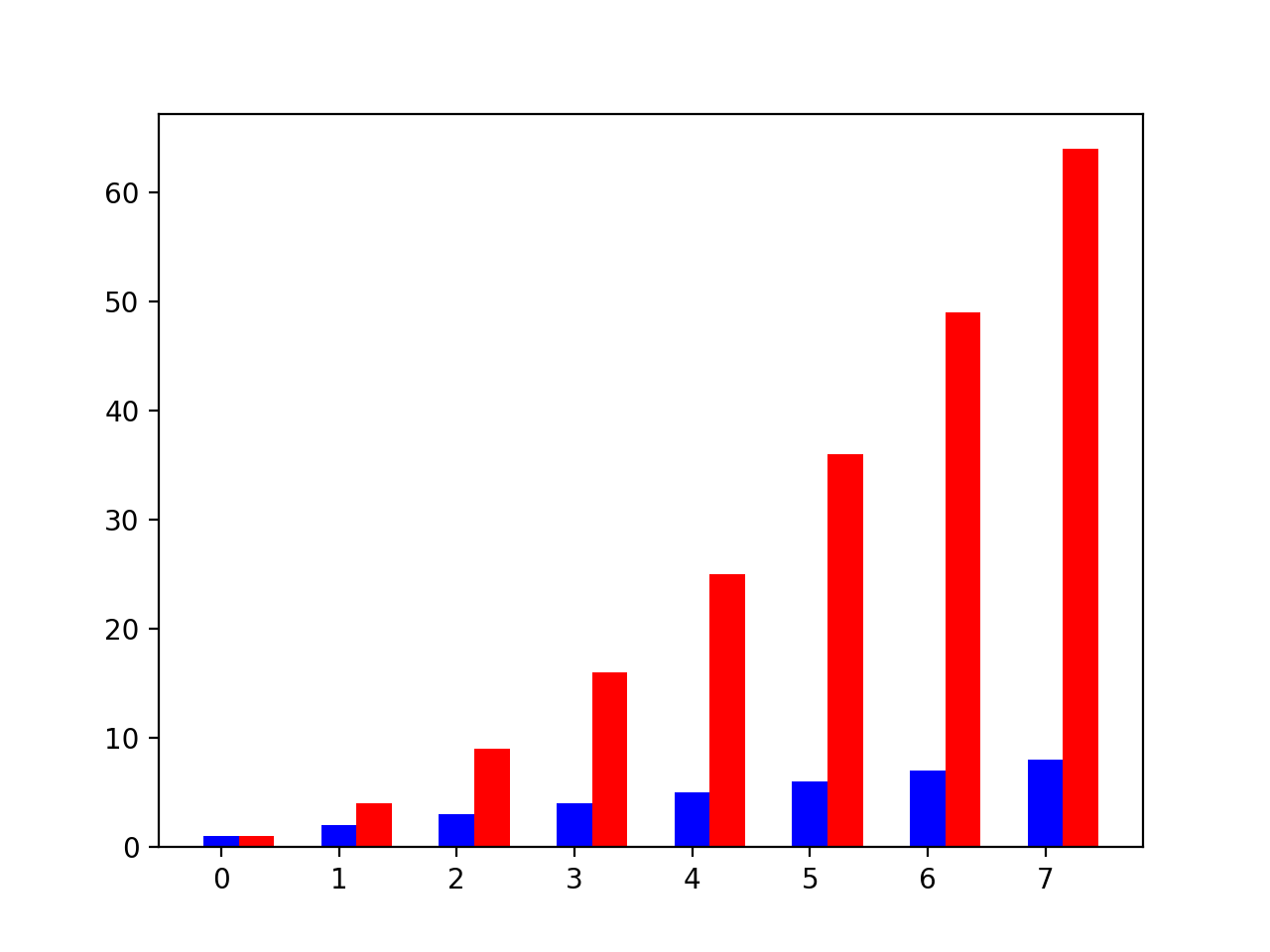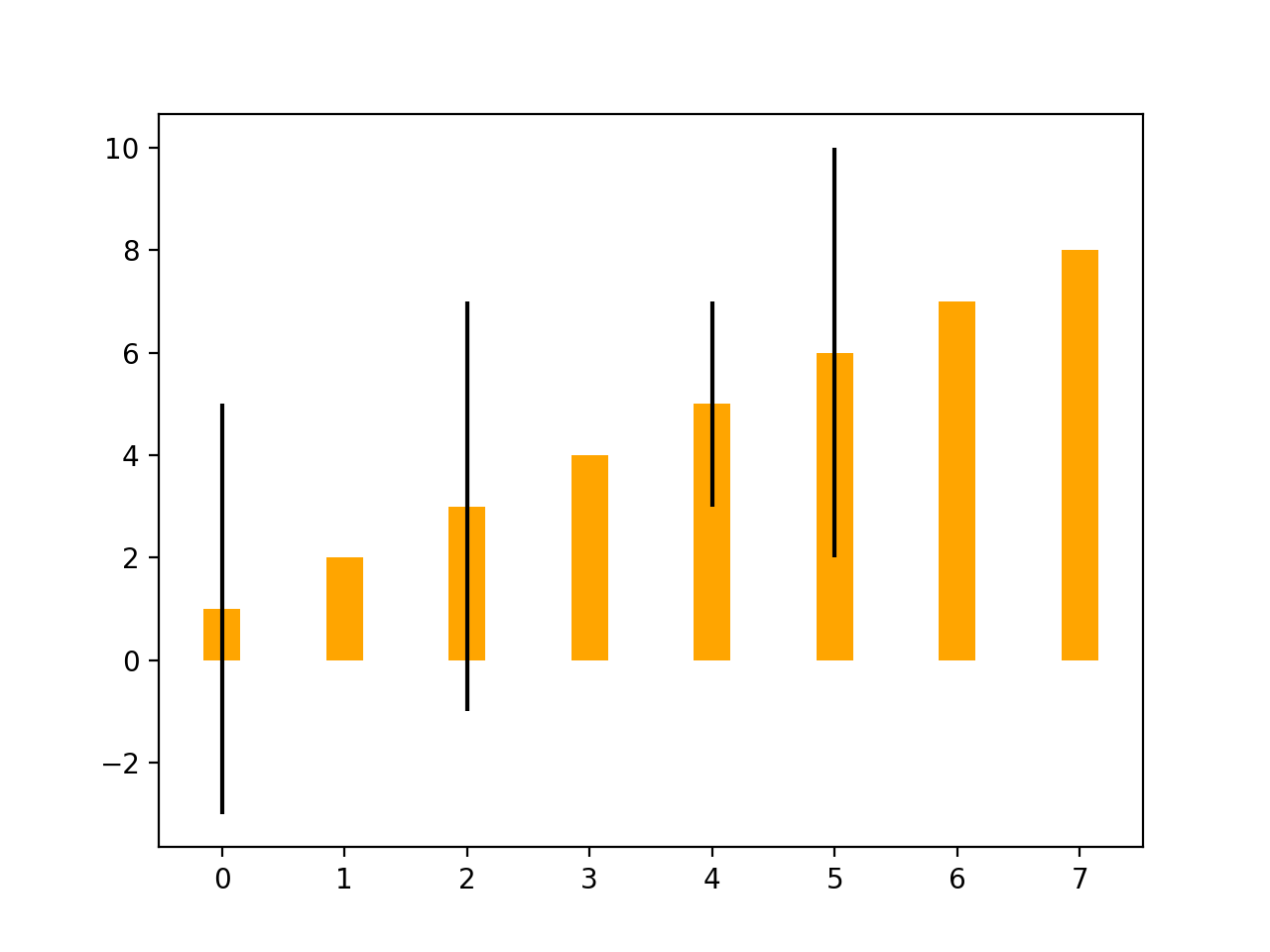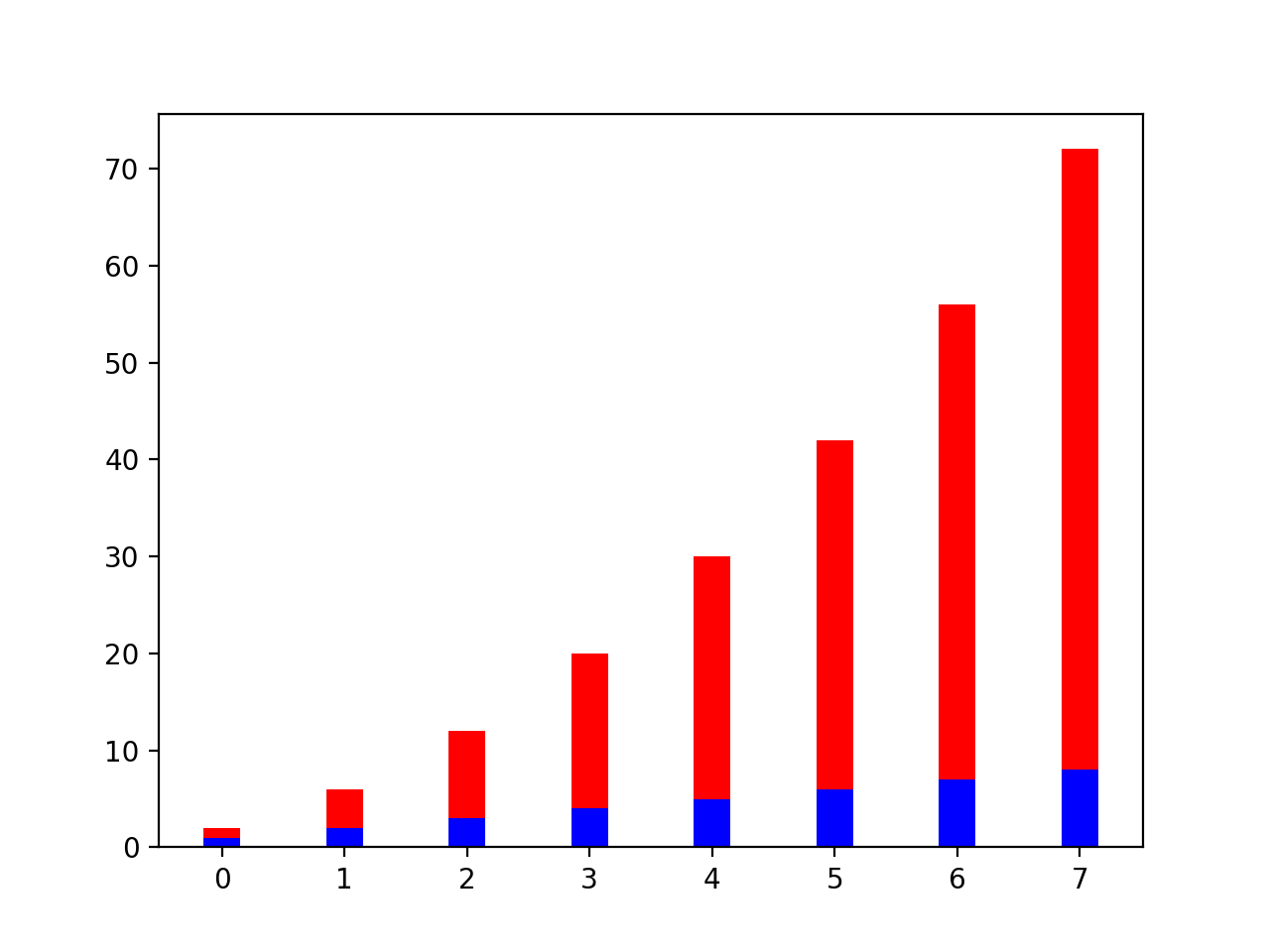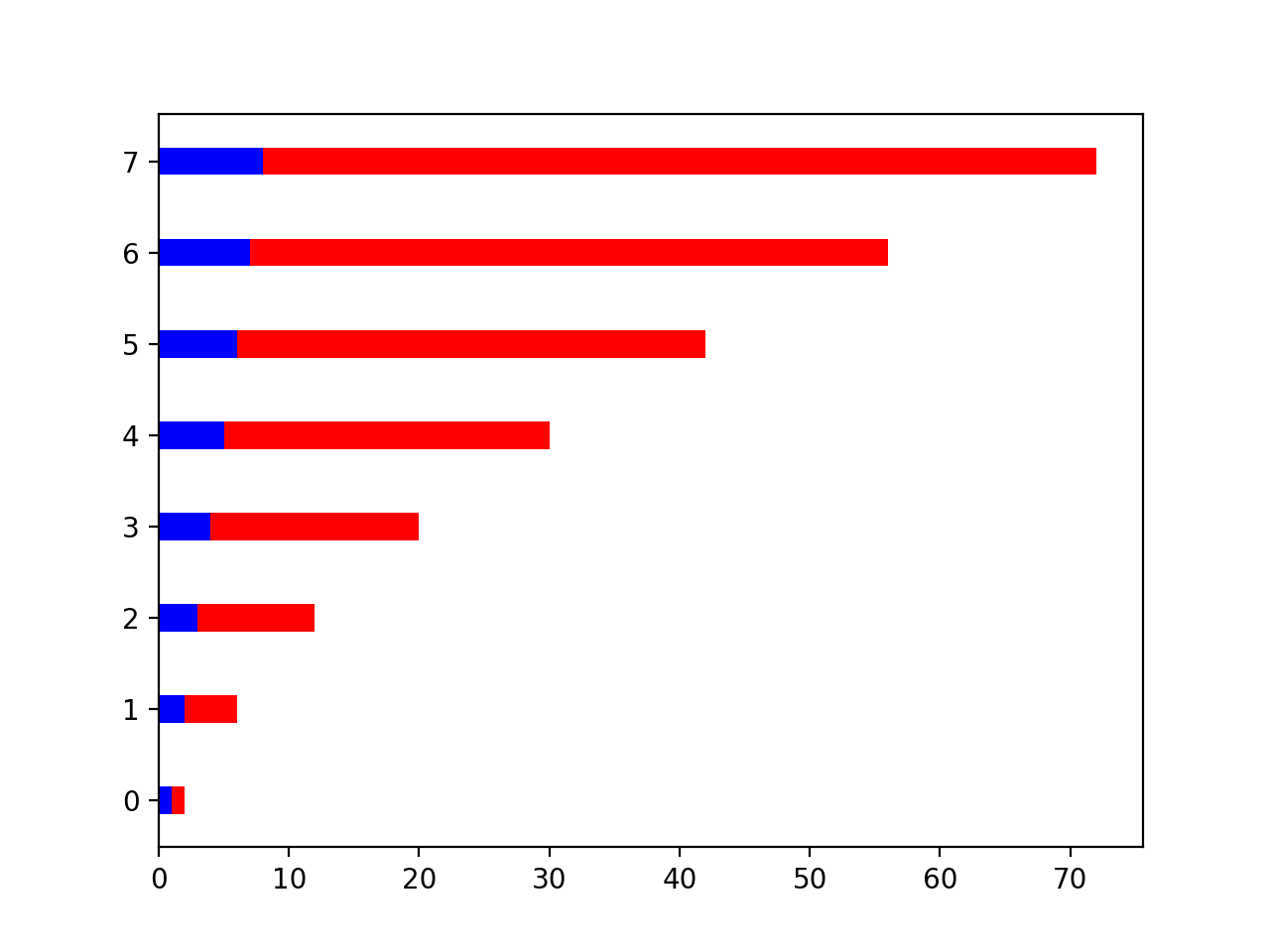# Bar Charts

%matplotlib notebook

import matplotlib.pyplot as plt
import numpy as np

linear_data = np.array([1,2,3,4,5,6,7,8])
exponential_data = linear_data**2

plt.figure()

xvals = range(8)
plt.bar(xvals,
linear_data,
width = 0.3,
color='blue');

<IPython.core.display.Javascript object>In order to make room for a side-by-side bar chart, for each original x-value, add a constant amount of space. Alignment of x-axis labels is one of the more cumbersome aspects of matplotlib.

Further, adding dates as x-axis labels must be completed manually. And, there is not an obvious way to plot several series of data in groups across time, for example.

new_xvals = []

for item in xvals:
new_xvals.append(item+0.3)

plt.bar(new_xvals,
exponential_data,
width = 0.3,
color='red');


Error bars can be added to the y-axis.

plt.figure()

from random import randint

linear_err = [randint(0,5) for x in range(len(linear_data))]
plt.bar(xvals,
linear_data,
width = 0.3,
color='orange',
yerr=linear_err);

<IPython.core.display.Javascript object>Stacked bar charts can be made by specifying the bottom attributes.

plt.figure()
xvals = range(len(linear_data))
plt.bar(xvals,
linear_data,
width = 0.3,
color='b')
plt.bar(xvals,
exponential_data,
width = 0.3,
bottom=linear_data,
color='r');

<IPython.core.display.Javascript object>Finally, horizontal bar charts can be made using barh instead of bar. Note that what had been the width parameter becomes the height parameter, and bottom becomes left.

plt.figure()
xvals = range(8)
plt.barh(xvals,
linear_data,
height = 0.3,
color='b')
plt.barh(xvals,
exponential_data,
height = 0.3,
left=linear_data,
color='r');

<IPython.core.display.Javascript object>